## 4. The natural logarithm.

Since the natural exponential function is defined over all ℜ and is always increasing, it is bijective and therefore invertible, that is, it exists a function, that we now call invexp(x), such that invexp(exp(x))=x.

The domain of invexp(x) coincides with the codomain of exp(x), that is, invexp(x) can be applied only to positive real numbers.

The function invexp(x) is commonly said natural logarithm and is denoted by ln (sometimes also by log).

So, by definition,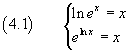If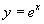then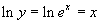, so the equalitiesand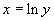are perfectly equivalent, they represent the same relation between x and y.

In particular, from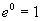we have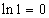and from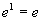we have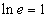.

### The logarithm derivative

From the second of (4.1) we have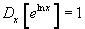By applying the derivation chain rule we have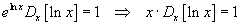and finally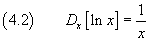### Basic logarithms properties.

The equalities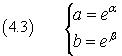are equivalent to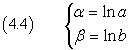By multiplying term by term the (4.3) we have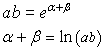and, from (4.4)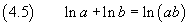The logarithm of a product equals the sum of the logarithms of its factors.

This property can be immediately extended to any number of factors.

Fromwe have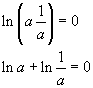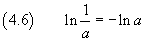The logarithm of the reciprocal of a number is the opposite of the logarithm of the number itself.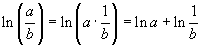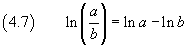The logarithm of a ratio of two numbers equals the difference between the logarithms of the numbers.

Given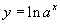we have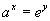and, raising both the terms to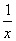,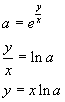Therefore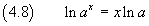The logarithm of a power is equal to the exponent times the logarithm of the base.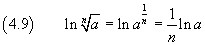The logarithm of a root equals the logarithm of the radicand divided by the index of the root.